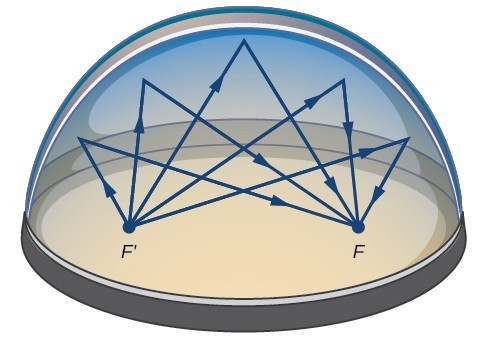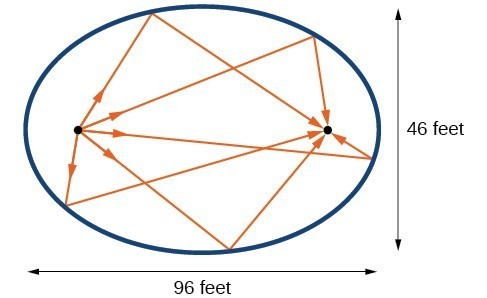## Solving Applied Problems Involving Ellipses

Many real-world situations can be represented by ellipses, including orbits of planets, satellites, moons and comets, and shapes of boat keels, rudders, and some airplane wings. A medical device called a lithotripter uses elliptical reflectors to break up kidney stones by generating sound waves. Some buildings, called whispering chambers, are designed with elliptical domes so that a person whispering at one focus can easily be heard by someone standing at the other focus. This occurs because of the acoustic properties of an ellipse. When a sound wave originates at one focus of a whispering chamber, the sound wave will be reflected off the elliptical dome and back to the other focus. In the whisper chamber at the Museum of Science and Industry in Chicago, two people standing at the foci—about 43 feet apart—can hear each other whisper.Figure 12

### Example 7: Locating the Foci of a Whispering Chamber

The Statuary Hall in the Capitol Building in Washington, D.C. is a whispering chamber. Its dimensions are 46 feet wide by 96 feet long as shown in Figure 13.

a. What is the standard form of the equation of the ellipse representing the outline of the room? Hint: assume a horizontal ellipse, and let the center of the room be the point $\left(0,0\right)$.

b. If two senators standing at the foci of this room can hear each other whisper, how far apart are the senators? Round to the nearest foot.Figure 13

### Solution

a. We are assuming a horizontal ellipse with center $\left(0,0\right)$, so we need to find an equation of the form $\frac{{x}^{2}}{{a}^{2}}+\frac{{y}^{2}}{{b}^{2}}=1$, where $a>b$. We know that the length of the major axis, $2a$, is longer than the length of the minor axis, $2b$. So the length of the room, 96, is represented by the major axis, and the width of the room, 46, is represented by the minor axis.
• Solving for $a$, we have $2a=96$, so $a=48$, and ${a}^{2}=2304$.
• Solving for $b$, we have $2b=46$, so $b=23$, and ${b}^{2}=529$.

Therefore, the equation of the ellipse is $\frac{{x}^{2}}{2304}+\frac{{y}^{2}}{529}=1$.

b. To find the distance between the senators, we must find the distance between the foci, $\left(\pm c,0\right)$, where ${c}^{2}={a}^{2}-{b}^{2}$. Solving for $c$, we have:
$\begin{array}{ll}{c}^{2}={a}^{2}-{b}^{2}\hfill & \hfill \\ {c}^{2}=2304 - 529\hfill & \begin{array}{cccc}& & & \end{array}\text{Substitute using the values found in part (a)}.\hfill \\ c=\pm \sqrt{2304 - 529}\hfill & \begin{array}{cccc}& & & \end{array}\text{Take the square root of both sides}.\hfill \\ c=\pm \sqrt{1775} \hfill & \begin{array}{cccc}& & & \end{array}\text{Subtract}.\hfill \\ c\approx \pm 42\hfill & \begin{array}{cccc}& & & \end{array}\text{Round to the nearest foot}.\hfill \end{array}$
The points $\left(\pm 42,0\right)$ represent the foci. Thus, the distance between the senators is $2\left(42\right)=84$ feet.

### Try It 7

Suppose a whispering chamber is 480 feet long and 320 feet wide.

a. What is the standard form of the equation of the ellipse representing the room? Hint: assume a horizontal ellipse, and let the center of the room be the point $\left(0,0\right)$.

b. If two people are standing at the foci of this room and can hear each other whisper, how far apart are the people? Round to the nearest foot.

Solution